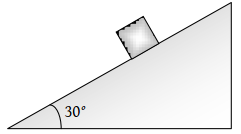A ball of mass m1 and another ball of mass m2 are dropped from equal height. If time taken by the balls are t1 and t2 respectively, then

1. ${t}_{1}=\frac{{t}_{2}}{2}$

2. ${t}_{1}={t}_{2}$

3. ${t}_{1}=4{t}_{2}$

4. ${t}_{1}=\frac{{t}_{2}}{4}$

Concept Questions :-

Uniformly accelerated motion
High Yielding Test Series + Question Bank - NEET 2020

Difficulty Level:

With what velocity a ball be projected vertically so that the distance covered by it in 5th second is twice the distance it covers in its 6th second $\left(g=10\text{\hspace{0.17em}}m/{s}^{2}\right)$

(1) 58.8 m/s

(2) 49 m/s

(3) 65 m/s

(4) 19.6 m/s

Concept Questions :-

Uniformly accelerated motion
High Yielding Test Series + Question Bank - NEET 2020

Difficulty Level:

A body sliding on a smooth inclined plane requires 4 seconds to reach the bottom starting from rest at the top. How much time does it take to cover one-fourth distance starting from rest at the top

(1) 1 s

(2) 2 s

(3) 4 s

(4) 16 s

Concept Questions :-

Uniformly accelerated motion
High Yielding Test Series + Question Bank - NEET 2020

Difficulty Level:

A ball is dropped downwards. After 1 second another ball is dropped downwards from the same point. What is the distance between them after 3 seconds

(1) 25 m

(2) 20 m

(3) 50 m

(4) 9.8 m

Concept Questions :-

Uniformly accelerated motion
High Yielding Test Series + Question Bank - NEET 2020

Difficulty Level:

A stone is thrown with an initial speed of 4.9 m/s from a bridge in vertically upward direction. It falls down in water after 2 sec. The height of the bridge is

(1) 4.9 m

(2) 9.8 m

(3) 19.8 m

(4) 24.7 m

Concept Questions :-

Uniformly accelerated motion
High Yielding Test Series + Question Bank - NEET 2020

Difficulty Level:

A stone is shot straight upward with a speed of 20 m/sec from a tower 200 m high. The speed with which it strikes the ground is approximately

(1) 60 m/sec

(2) 65 m/sec

(3) 70 m/sec

(4) 75 m/sec

Concept Questions :-

Uniformly accelerated motion
High Yielding Test Series + Question Bank - NEET 2020

Difficulty Level:

A body freely falling from the rest has a velocity ‘v’ after it falls through a height ‘h’. The distance it has to fall down for its velocity to become double, is

(1) 2 h

(2) 4 h

(3) 6 h

(4) 8 h

High Yielding Test Series + Question Bank - NEET 2020

Difficulty Level:

The time taken by a block of wood (initially at rest) to slide down a smooth inclined plane 9.8 m long (angle of inclination is ${30}^{o}$) is(1) $\frac{1}{2}\mathrm{sec}$

(2) 2 sec

(3) 4 sec

(4) 1 sec

Concept Questions :-

Uniformly accelerated motion
High Yielding Test Series + Question Bank - NEET 2020

Difficulty Level:

Velocity of a body on reaching the point from which it was projected upwards, is

1. $v=0$

2. $v=2u$

3. $v=0.5u$

4. $v=-u$

Concept Questions :-

Uniformly accelerated motion
High Yielding Test Series + Question Bank - NEET 2020

Difficulty Level:

A body projected vertically upwards with a velocity u returns to the starting point in 4 seconds. If g = 10m/sec2, the value of u is

(1) 5 m/sec

(2) 10 m/sec

(3) 15 m/sec

(4) 20 m/sec

Concept Questions :-

Uniformly accelerated motion